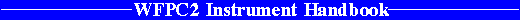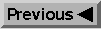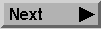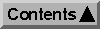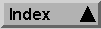System Throughput and SNR / Exposure Time Estimation

# 6.6 Exposure Time Estimation

In many instances one desires a certain SNR, and wishes to solve for the corresponding exposure time. Given the SNR, Equations 6.6, 6.7, or 6.14 can be solved for the exposure time, t. Since there are time-dependent and time-independent noise sources, quadratic equations are obtained. For example, we may solve equation 6.6 for the point source exposure time: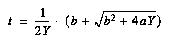where the term A contains the time-independent noise sources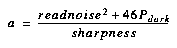and B contains the time-dependent noise sources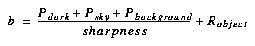and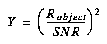Equations for aperture photometry (6.7) and extended sources (6.12) can be solved with similar results. Parameters are as described in Tables 6.6, 6.8, and 6.9. We again note that the on-line WFPC2 Exposure Time Calculator program provides an easy method for these calculations.